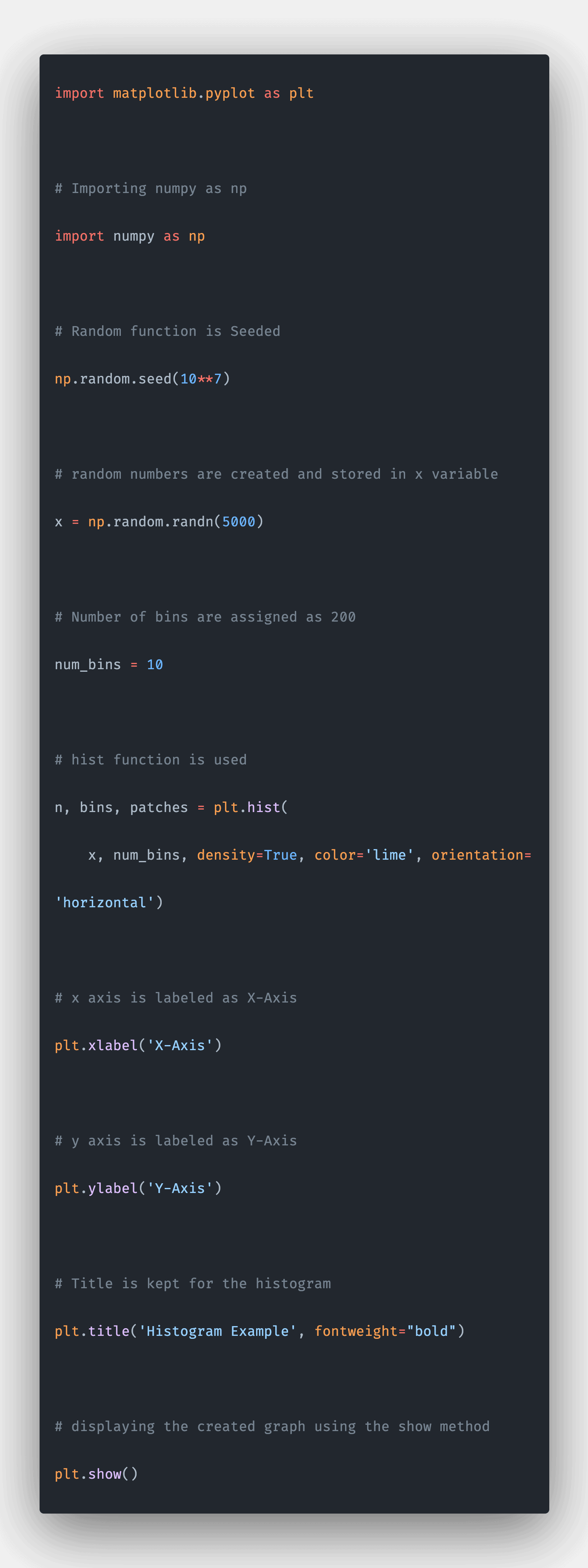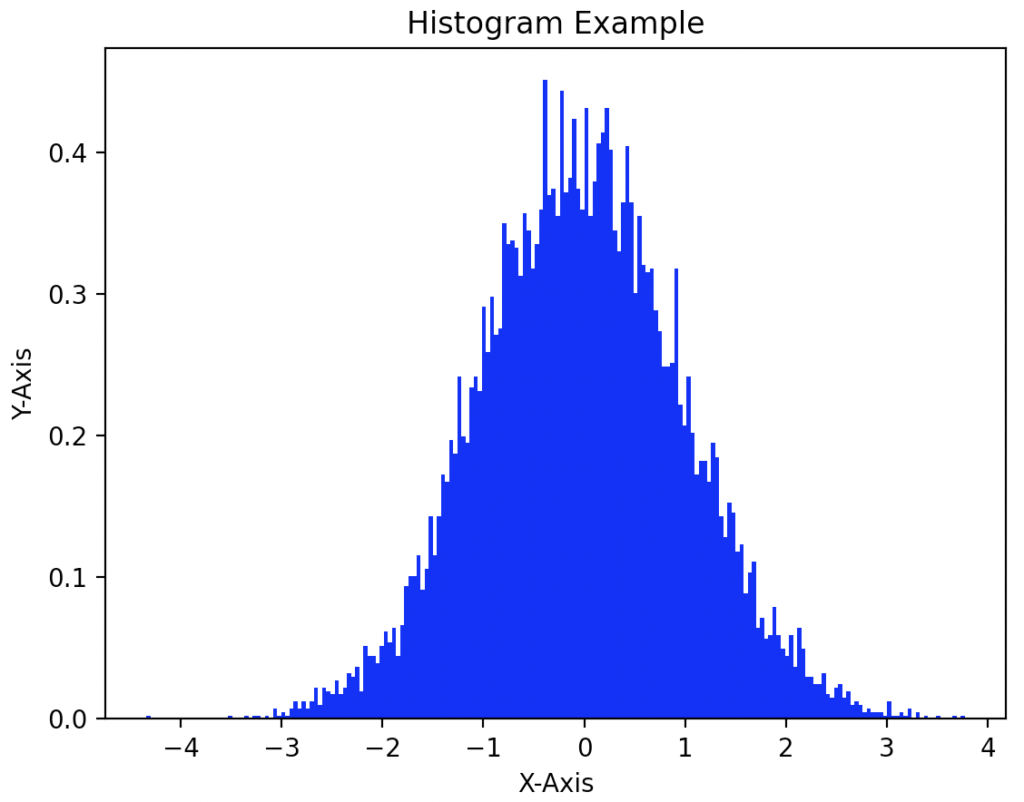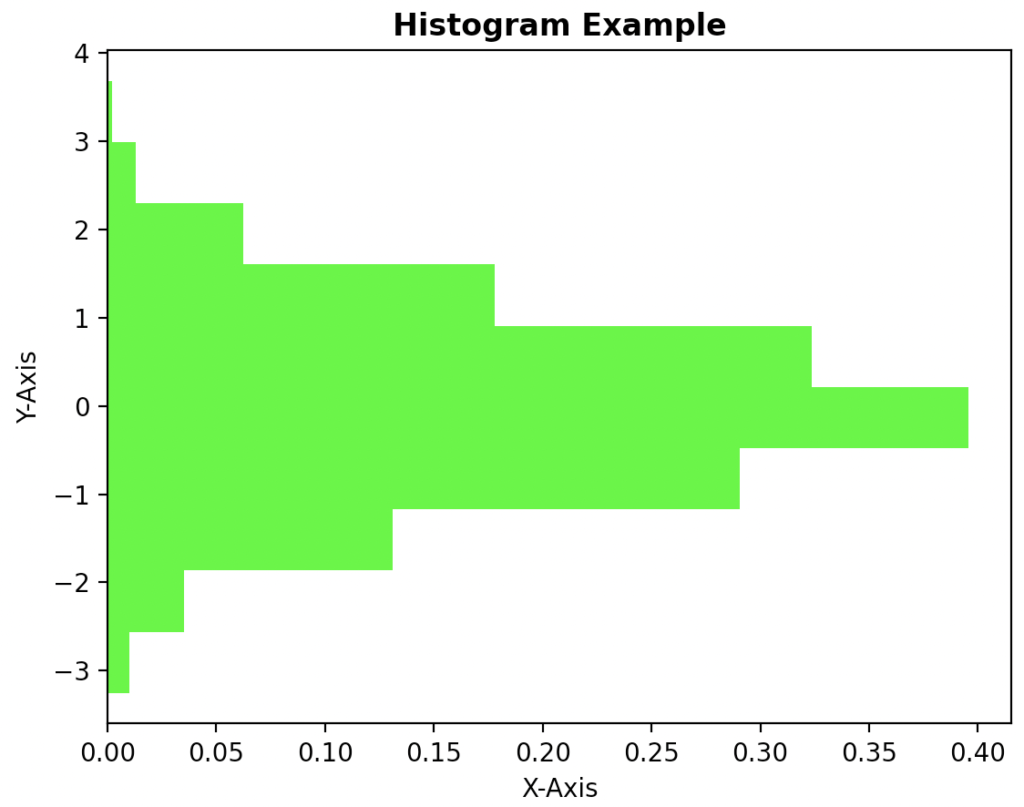# Matplotlib histogram: The Complete Guide

0
2Python Matplotlib library assists in visualizing and researching the data and helps in better understanding of the data with the help of graphical visualizations that can be simulated using the matplotlib library.

## Matplotlib histogram

The matplotlib.pyplot.hist() is a built-in matplotlib library function that creates a histogram for a given point and displays the graph as the output.

### Syntax

``````matplotlib.pyplot.hist(x, bins=None, range=None, density=False,
weights=None, cumulative=False, bottom=None, histtype='bar',
align='mid', orientation='vertical', rwidth=None, log=False,
color=None, label=None, stacked=False, *, data=None, **kwargs) ``````

### Parameters

The matplotlib.pyplot.hist() function has one required argument as parameter:

1. x: This is the required argument. This argument takes an array as a value. This array consists of the points.
2. bins:  This argument takes an integer or sequence of integers as value. This argument is optional. By default, it is assigned as 10.
3. range: This is an optional argument. If the lower value and upper value are provided in the range argument, then the values less than the lower value and greater than the upper value will not be plotted in the graph. By default, this value is provided with minimum values for the lower value and maximum for the upper value.
4. density: This takes a Boolean value. If this value is passed as True, then the probability density function is returned. By default, this is passed as False. data: An object with labeled data is passed as an argument. This is an optional argument.
5. weights: This is an optional argument. This argument can be passed with an array of values. This array’s shape should be equal to the shape of the x array.
6. cumulative: This is an optional argument. This argument takes a Boolean value as a value to the argument. If this is passed as True, then each bin value is added with the value of the previous bin, and the total score is kept as the value for the bin.
7. bottom: This is an optional argument. This argument takes values in an array. This array consists of locations where the bin is to be located.
8. histtype: This is an optional argument. This argument takes string values. This represents the type of histogram that is needed to be plotted. The values can be bar, barstacked, step, or stepfilled.
9. align: This argument represents the alignment of the graph. This has values like left, right, and mid.
10. orientation: This value describes the orientation of the graph. This has values like vertical and horizontal. By default, it is kept vertical.
11. rwidth: It is the relative width of the bars. By default, it is kept as None.
12. log: It takes a Boolean value as an argument. If this is True, then the scale is set to log scale.
13. color: The colors for the graph is passed in this argument. The colors can also be passed in the array.
14. label: This argument string as inputs. If multiple datasets are used at that stage, the first data gets the label passed in this argument. Other data gets the value provided in the legend.
15. stacked: It takes a Boolean value as a value to this argument. If true, then multiple values are stacked on top of each other.

### Return value

The matplotlib.pyplot.hist() function returns an array of n elements. Then it returns the bins array. It also returns the patches array.

## Program for creating a histogram using matplotlib.pyplot.hist

``````# Importing matplotlib.pyplot as plt.
import matplotlib.pyplot as plt

# Importing numpy as np
import numpy as np

# Random function is Seeded
np.random.seed(10**7)

# Random numbers are created and stored in x variable
x = np.random.randn(10000)

# Number of bins are assigned as 200
num_bins = 200

# Hist function is used
n, bins, patches = plt.hist(x, num_bins, density=2, color='blue')

# X-axis is labeled as X-Axis
plt.xlabel('X-Axis')

# y axis is labeled as Y-Axis
plt.ylabel('Y-Axis')

# Title is kept for the histogram
plt.title('Histogram Example')

# displaying the created graph using the show method
plt.show()``````

#### OutputIn this program, we imported matplotlib.pyplot for plotting the histogram. The matplotlib library consists of all the functions for plotting different types of graphs. Then, we imported numpy for creating x coordinates.

Then, we created a random array and stored it in x. Then we have assigned the number of bins to 200.

Then, we used the hist() function. First, the x array is passed as the required argument, then we have given several bins as 200, then we have given density as 2 and color as blue. This function returns three arrays.

## Program for creating a histogram using matplotlib.pyplot.hist

``````# Importing matplotlib.pyplot as plt.
import matplotlib.pyplot as plt

# Importing numpy as np
import numpy as np

# Random function is Seeded
np.random.seed(10**7)

# random numbers are created and stored in x variable
x =np.random.randn(5000)

# Number of bins are assigned as 200
num_bins = 10

# hist function is used
n, bins, patches = plt.hist(x, num_bins,density = True,color ='lime',orientation='horizontal')

# x axis is labeled as X-Axis
plt.xlabel('X-Axis')

# y axis is labeled as Y-Axis
plt.ylabel('Y-Axis')

# Title is kept for the histogram
plt.title('Histogram Example',fontweight ="bold")

# displaying the created graph using the show method
plt.show()
``````

#### OutputIn this program, we imported matplotlib.pyplot for plotting the histogram. The matplotlib library consists of all the functions for plotting different types of graphs.

Then we imported numpy for creating x coordinates. Then we created a random array and stored it in x. Then, we assigned the number of bins as 10.

Then we called the hist() function. First, the x array is passed as the required argument, then we have passed a number of bins as 10 then we passed density as True then we have passed the orientation as horizontal, and then color as lime. The hist() function returns three arrays.

This site uses Akismet to reduce spam. Learn how your comment data is processed.